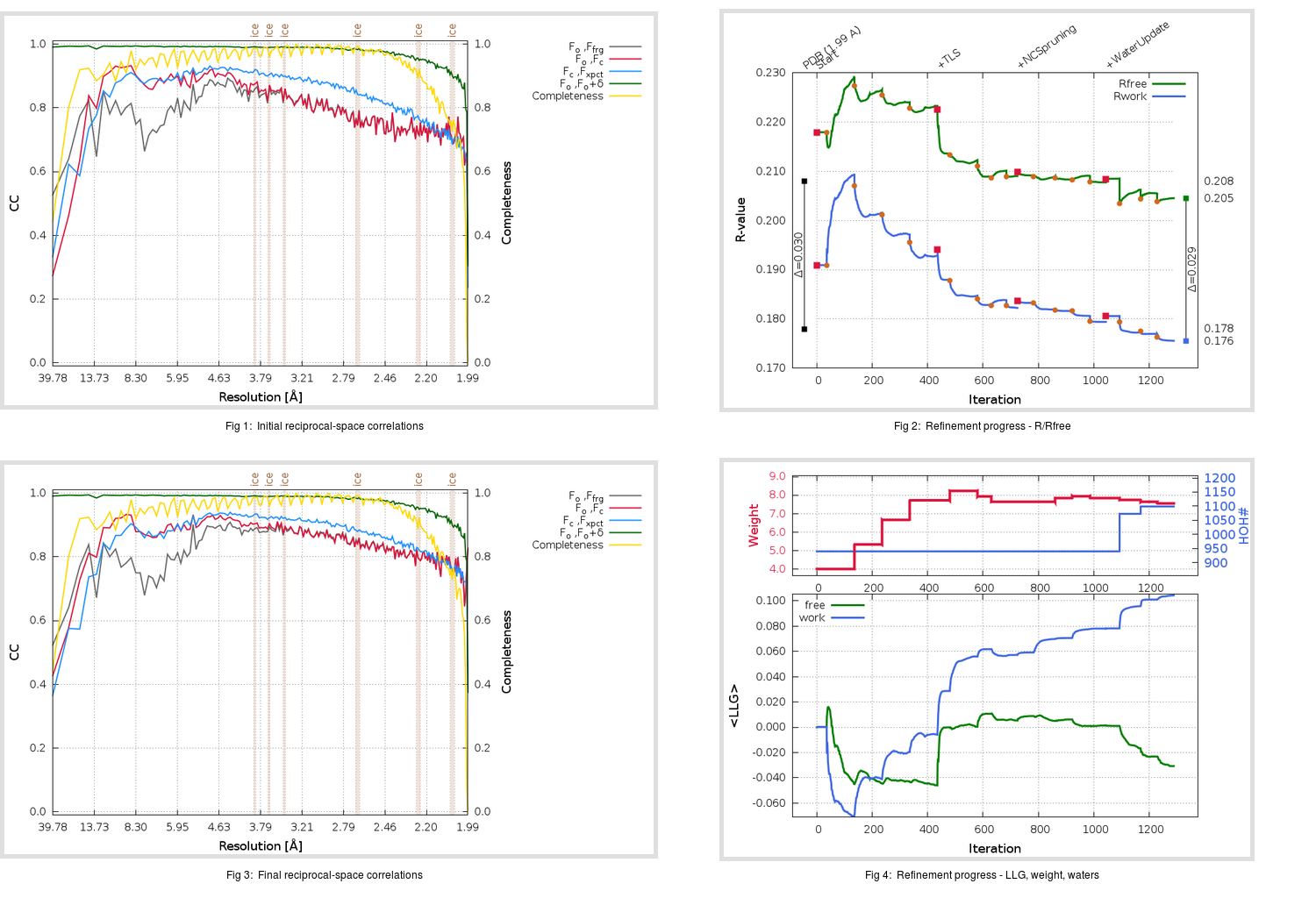Content:

```    Diffraction limits & principal axes of ellipsoid fitted to diffraction cut-off surface:
1.963         0.9869   0.0000   0.1616       0.983 a* - 0.186 c*
1.928         0.0000   1.0000   0.0000       b*
2.185        -0.1616   0.0000   0.9869      -0.162 a* + 0.987 c*
```

## Deposited

` `
 Date deposited Date data collection Resolution R, Rfree 20180119 20150624 1.99 0.1760 0.2080

Molprobity (CCP4 7.0 version) summary:

```Ramachandran outliers =   0.00 %
favored =  97.57 %
Rotamer outliers      =   3.59 %
C-beta deviations     =     0
Clashscore            =   5.67
RMS(bonds)            =   0.0062
RMS(angles)           =   1.11
MolProbity score      =   1.82
Resolution            =   1.99
R-work                =   0.1760
R-free                =   0.2080
```

```Number of waters      =   940

<B> (all atoms) =   40.56 ( sd =   14.52 ) for      13929 non-hydrogen atoms
<B>   (protein) =   40.30 ( sd =   14.78 ) for      12989 non-hydrogen atoms
<B>     (water) =   44.11 ( sd =    9.52 ) for        940 non-hydrogen atoms
<B>    (others) =    0.00 ( sd =    0.00 ) for          0 non-hydrogen atoms

B min/max       (all non-hydrogen atoms) =   14.07 /  142.98
B min/max   (protein non-hydrogen atoms) =   14.07 /  142.98
B min/max     (water non-hydrogen atoms) =   19.31 /   72.92
B min/max     (other non-hydrogen atoms) =    0.00 /    0.00
```

## BUSTER (re-)refinement

` `

Molprobity (CCP4 7.0 version) summary:

```Ramachandran outliers =   0.00 %
favored =  97.51 %
Rotamer outliers      =   3.12 %
C-beta deviations     =     0
Clashscore            =   4.22
RMS(bonds)            =   0.0115
RMS(angles)           =   1.59
MolProbity score      =   1.68
Resolution            =   1.99
R-work                =   0.1756
R-free                =   0.2046
```

```Number of waters      =  1099

<B> (all atoms) =   39.75 ( sd =   12.17 ) for      14088 non-hydrogen atoms
<B>   (protein) =   39.02 ( sd =   11.99 ) for      12989 non-hydrogen atoms
<B>     (water) =   48.32 ( sd =   11.03 ) for       1099 non-hydrogen atoms
<B>    (others) =    0.00 ( sd =    0.00 ) for          0 non-hydrogen atoms

B min/max       (all non-hydrogen atoms) =   17.84 /   96.20
B min/max   (protein non-hydrogen atoms) =   17.84 /   95.18
B min/max     (water non-hydrogen atoms) =   19.16 /   96.20
B min/max     (other non-hydrogen atoms) =    0.00 /    0.00
```

Refinement progression:Results:

` `
 File Remark 6C6X_aB_refine.01_04_refine.pdb.gz exact refinement commands are in header 6C6X_aB_refine.01_04_refine.mtz.gz including original deposited data and several re-refinement map coefficients 6C6X_aB_refine.01_04_BUSTER_model.cif.gz including any non-standard compound restraints 6C6X_aB_refine.01_04_BUSTER_refln.cif.gz